## Kronecker Delta

The simplest interpretation of the Kronecker delta is as the discrete version of the Delta Function defined by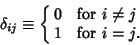(1)

It has the Complex Generating Function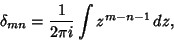(2)

whereandare Integers. In 3-space, the Kronecker delta satisfies the identities(3)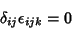(4)(5)(6)

where Einstein Summation is implicitly assumed,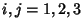, and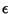is the Permutation Symbol.

Technically, the Kronecker delta is a Tensor defined by the relationship(7)

Since, by definition, the coordinatesand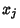are independent for,(8)

so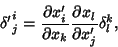(9)

and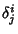is really a mixed second Rank Tensor. It satisfies(10)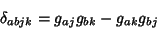(11)(12)